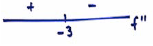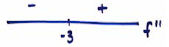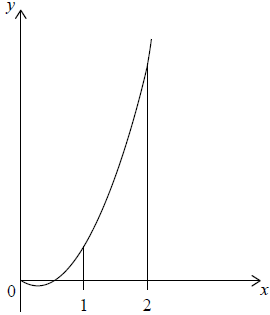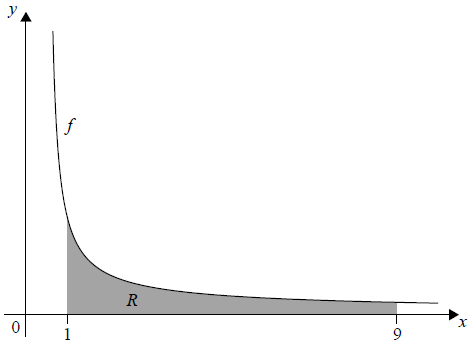# IBDP Maths AA: Topic SL 5.10: Indefinite integral: IB style Questions SL Paper 1

### Question

The graph of a function f passes through the point (ln4, 20).

Given that f ′(x) = 6e2x , find f (x).

Ans:

evidence of integration

eg $$\int f'(x)dx, \int 6e^{2x}$$

correct integration (accept missing + c)

eg $$\frac{1}{2}\times 6e^{2x}, 3e^{2x}$$ +c

substituting initial condition into their integrated expression (must have +c )

eg $$3e^{2\times ln4}$$+c = 20

correct application of log ($$a^{b})$$= b log a rule (seen anywhere)

eg 2ln4 = ln16, eln16, ln42

correct application of  elna= a rule (seen anywhere)

eg $$e^{ln16}= 16, (e^{ln4})^{2}=4^{2}$$

correct working

eg $$3\times 16+c= 20, 3\times (4^{2})+c= 20, c=20 c=-28$$

$$f(x)=3e^{2x}- 28$$

### Question

Let $$f(x) = \int {\frac{{12}}{{2x – 5}}} {\rm{d}}x$$ , $$x > \frac{5}{2}$$ . The graph of $$f$$ passes through ($$4$$, $$0$$) .

Find $$f(x)$$ .

## Markscheme

attempt to integrate which involves $$\ln$$     (M1)

eg   $$\ln (2x – 5)$$ , $$12\ln 2x – 5$$ , $$\ln 2x$$

correct expression (accept absence of $$C$$)

eg   $$12\ln (2x – 5)\frac{1}{2} + C$$ , $$6\ln (2x – 5)$$     A2

attempt to substitute (4,0) into their integrated     (M1)

eg $$0 = 6\ln (2 \times 4 – 5)$$ , $$0 = 6\ln (8 – 5) + C$$

$$C = – 6\ln 3$$     (A1)

$$f(x) = 6\ln (2x – 5) – 6\ln 3$$ $$\left( { = 6\ln \left( {\frac{{2x – 5}}{3}} \right)} \right)$$ (accept $$6\ln (2x – 5) – \ln {3^6}$$ )     A1     N5

Note: Exception to the FT rule. Allow full FT on incorrect integration which must involve $$\ln$$.

[6 marks]

## Question

A function f (x) has derivative f ′(x) = 3x2 + 18x. The graph of f has an x-intercept at x = −1.

Find f (x).


a.

The graph of f has a point of inflexion at x = p. Find p.


b.

Find the values of x for which the graph of f is concave-down.


c.

## Markscheme

evidence of integration       (M1)

eg  $$\int {f’\left( x \right)}$$

correct integration (accept absence of C)       (A1)(A1)

eg  $${x^3} + \frac{{18}}{2}{x^2} + C,\,\,{x^3} + 9{x^2}$$

attempt to substitute x = −1 into their = 0 (must have C)      M1

eg  $${\left( { – 1} \right)^3} + 9{\left( { – 1} \right)^2} + C = 0,\,\, – 1 + 9 + C = 0$$

Note: Award M0 if they substitute into original or differentiated function.

correct working       (A1)

eg  $$8 + C = 0,\,\,\,C = – 8$$

$$f\left( x \right) = {x^3} + 9{x^2} – 8$$      A1 N5

[6 marks]

a.

METHOD 1 (using 2nd derivative)

recognizing that f” = 0 (seen anywhere)      M1

correct expression for f”      (A1)

eg   6x + 18, 6p + 18

correct working      (A1)

6+ 18 = 0

p = −3       A1 N3

METHOD 1 (using 1st derivative)

recognizing the vertex of f′ is needed       (M2)

eg   $$– \frac{b}{{2a}}$$ (must be clear this is for f′)

correct substitution      (A1)

eg   $$\frac{{ – 18}}{{2 \times 3}}$$

p = −3       A1 N3

[4 marks]

b.

valid attempt to use f” (x) to determine concavity      (M1)

eg   f” (x) < 0, f” (−2), f” (−4),  6x + 18 ≤ 0correct working       (A1)

eg   6x + 18 < 0, f” (−2) = 6, f” (−4) = −6f concave down for x < −3 (do not accept ≤ −3)       A1 N2

[3 marks]

c.

## Question

Let $$f\left( x \right) = 6{x^2} – 3x$$. The graph of $$f$$ is shown in the following diagram.Find $$\int {\left( {6{x^2} – 3x} \right){\text{d}}x}$$.


a.

Find the area of the region enclosed by the graph of $$f$$, the x-axis and the lines x = 1 and x = 2 .


b.

## Markscheme

$$2{x^3} – \frac{{3{x^2}}}{2} + c\,\,\,\left( {{\text{accept}}\,\,\frac{{6{x^3}}}{3} – \frac{{3{x^2}}}{2} + c} \right)$$     A1A1 N2

Notes: Award A1A0 for both correct terms if +c is omitted.
Award A1A0 for one correct term eg $$2{x^3} + c$$.
Award A1A0 if both terms are correct, but candidate attempts further working to solve for c.

[2 marks]

a.

substitution of limits or function (A1)

eg  $$\int_1^2 {f\left( x \right)} \,{\text{d}}x,\,\,\left[ {2{x^3} – \frac{{3{x^2}}}{2}} \right]_1^2$$

substituting limits into their integrated function and subtracting     (M1)

eg  $$\frac{{6 \times {2^3}}}{3} – \frac{{3 \times {2^2}}}{2} – \left( {\frac{{6 \times {1^3}}}{3} + \frac{{3 \times {1^2}}}{2}} \right)$$

Note: Award M0 if substituted into original function.

correct working      (A1)

eg  $$\frac{{6 \times 8}}{3} – \frac{{3 \times 4}}{2} – \frac{{6 \times 1}}{3} + \frac{{3 \times 1}}{2},\,\,\left( {16 – 6} \right) – \left( {2 – \frac{3}{2}} \right)$$

$$\frac{{19}}{2}$$     A1 N3

[4 marks]

b.

## Question

Given that $$\int_0^5 {\frac{2}{{2x + 5}}} {\rm{d}}x = \ln k$$ , find the value of k .

## Markscheme

correct integration, $$2 \times \frac{1}{2}\ln (2x + 5)$$     A1A1

Note: Award A1 for $$2 \times \frac{1}{2}( = 1)$$ and A1 for $$\ln (2x + 5)$$ .

evidence of substituting limits into integrated function and subtracting     (M1)

e.g. $$\ln (2 \times 5 + 5) – \ln (2 \times 0 + 5)$$

correct substitution     A1

e.g. $$\ln 15 – \ln 5$$

correct working     (A1)

e.g. $$\ln \frac{{15}}{5},\ln 3$$

$$k = 3$$     A1     N3

[6 marks]

## Question

Let $$f(x) = \int {\frac{{12}}{{2x – 5}}} {\rm{d}}x$$ , $$x > \frac{5}{2}$$ . The graph of $$f$$ passes through ($$4$$, $$0$$) .

Find $$f(x)$$ .

## Markscheme

attempt to integrate which involves $$\ln$$     (M1)

eg   $$\ln (2x – 5)$$ , $$12\ln 2x – 5$$ , $$\ln 2x$$

correct expression (accept absence of $$C$$)

eg   $$12\ln (2x – 5)\frac{1}{2} + C$$ , $$6\ln (2x – 5)$$     A2

attempt to substitute (4,0) into their integrated     (M1)

eg $$0 = 6\ln (2 \times 4 – 5)$$ , $$0 = 6\ln (8 – 5) + C$$

$$C = – 6\ln 3$$     (A1)

$$f(x) = 6\ln (2x – 5) – 6\ln 3$$ $$\left( { = 6\ln \left( {\frac{{2x – 5}}{3}} \right)} \right)$$ (accept $$6\ln (2x – 5) – \ln {3^6}$$ )     A1     N5

Note: Exception to the FT rule. Allow full FT on incorrect integration which must involve $$\ln$$.

[6 marks]

## Question

Let  $$f:x \mapsto {\sin ^3}x$$ .

(i) Write down the range of the function f .

(ii) Consider $$f(x) = 1$$ , $$0 \le x \le 2\pi$$ . Write down the number of solutions to this equation. Justify your answer.


a.

Find $$f'(x)$$ , giving your answer in the form $$a{\sin ^p}x{\cos ^q}x$$ where $$a{\text{, }}p{\text{, }}q \in \mathbb{Z}$$ .


b.

Let $$g(x) = \sqrt 3 \sin x{(\cos x)^{\frac{1}{2}}}$$ for $$0 \le x \le \frac{\pi }{2}$$ . Find the volume generated when the curve of g is revolved through $$2\pi$$ about the x-axis.


c.

## Markscheme

(i) range of f is $$[ – 1{\text{, }}1]$$ , $$( – 1 \le f(x) \le 1)$$     A2     N2

(ii) $${\sin ^3}x \Rightarrow 1 \Rightarrow \sin x = 1$$     A1

justification for one solution on $$[0{\text{, }}2\pi ]$$    R1

e.g. $$x = \frac{\pi }{2}$$ , unit circle, sketch of $$\sin x$$

1 solution (seen anywhere)     A1     N1

[5 marks]

a.

$$f'(x) = 3{\sin ^2}x\cos x$$     A2     N2

[2 marks]

b.

using $$V = \int_a^b {\pi {y^2}{\rm{d}}x}$$     (M1)

$$V = \int_0^{\frac{\pi }{2}} {\pi (\sqrt 3 } \sin x{\cos ^{\frac{1}{2}}}x{)^2}{\rm{d}}x$$     (A1)

$$= \pi \int_0^{\frac{\pi }{2}} {3{{\sin }^2}x\cos x{\rm{d}}x}$$     A1

$$V = \pi \left[ {{{\sin }^3}x} \right]_0^{\frac{\pi }{2}}$$ $$\left( { = \pi \left( {{{\sin }^3}\left( {\frac{\pi }{2}} \right) – {{\sin }^3}0} \right)} \right)$$     A2

evidence of using $$\sin \frac{\pi }{2} = 1$$ and $$\sin 0 = 0$$     (A1)

e.g. $$\pi \left( {1 – 0} \right)$$

$$V = \pi$$     A1     N1

[7 marks]

c.

## Question

Let $$f(x) = \frac{{2x}}{{{x^2} + 5}}$$.

Use the quotient rule to show that $$f'(x) = \frac{{10 – 2{x^2}}}{{{{({x^2} + 5)}^2}}}$$.


a.

Find $$\int {\frac{{2x}}{{{x^2} + 5}}{\text{d}}x}$$.


b.

The following diagram shows part of the graph of $$f$$.The shaded region is enclosed by the graph of $$f$$, the $$x$$-axis, and the lines $$x = \sqrt 5$$ and $$x = q$$. This region has an area of $$\ln 7$$. Find the value of $$q$$.


c.

## Markscheme

derivative of $$2x$$ is $$2$$ (must be seen in quotient rule)     (A1)

derivative of $${x^2} + 5$$ is $$2x$$ (must be seen in quotient rule)     (A1)

correct substitution into quotient rule     A1

eg     $$\frac{{({x^2} + 5)(2) – (2x)(2x)}}{{{{({x^2} + 5)}^2}}},{\text{ }}\frac{{2({x^2} + 5) – 4{x^2}}}{{{{({x^2} + 5)}^2}}}$$

eg    $$\frac{{2{x^2} + 10 – 4{x^2}}}{{{{({x^2} + 5)}^2}}},{\text{ }}\frac{{2{x^2} + 10 – 4{x^2}}}{{{x^4} + 10{x^2} + 25}}$$

$$f'(x) = \frac{{10 – 2{x^2}}}{{{{({x^2} + 5)}^2}}}$$     AG     N0

[4 marks]

a.

valid approach using substitution or inspection     (M1)

eg     $$u = {x^2} + 5,{\text{ d}}u = 2x{\text{d}}x,{\text{ }}\frac{1}{2}\ln ({x^2} + 5)$$

$$\int {\frac{{2x}}{{{x^2} + 5}}{\text{d}}x = \int {\frac{1}{u}{\text{d}}u} }$$     (A1)

$$\int {\frac{1}{u}{\text{d}}u = \ln u + c}$$     (A1)

$$\ln ({x^2} + 5) + c$$     A1     N4

[4 marks]

b.

correct expression for area     (A1)

eg     $$\left[ {\ln \left( {{x^2} + 5} \right)} \right]_{\sqrt 5 }^q,{\text{ }}\int\limits_{\sqrt 5 }^q {\frac{{2x}}{{{x^2} + 5}}{\text{d}}x}$$

substituting limits into their integrated function and subtracting (in either order)     (M1)

eg     $$\ln ({q^2} + 5) – \ln \left( {{{\sqrt 5 }^2} + 5} \right)$$

correct working     (A1)

eg     $$\ln \left( {{q^2} + 5} \right) – \ln 10,{\text{ }}\ln \frac{{{q^2} + 5}}{{10}}$$

equating their expression to $$\ln 7$$ (seen anywhere)     (M1)

eg     $$\ln \left( {{q^2} + 5} \right) – \ln 10 = \ln 7,{\text{ }}\ln \frac{{{q^2} + 5}}{{10}} = \ln 7,{\text{ }}\ln ({q^2} + 5) = \ln 7 + \ln 10$$

correct equation without logs     (A1)

eg     $$\frac{{{q^2} + 5}}{{10}} = 7,{\text{ }}{q^2} + 5 = 70$$

$${q^2} = 65$$     (A1)

$$q = \sqrt {65}$$     A1     N3

Note: Award A0 for $$q = \pm \sqrt {65}$$.

[7 marks]

c.

## Question

The following diagram shows the graph of $$f(x) = \frac{x}{{{x^2} + 1}}$$, for $$0 \le x \le 4$$, and the line $$x = 4$$.Let $$R$$ be the region enclosed by the graph of $$f$$ , the $$x$$-axis and the line $$x = 4$$.

Find the area of $$R$$.

## Markscheme

substitution of limits or function     (A1)

eg$$\;\;\;A = \int_0^4 {f(x),{\text{ }}\int {\frac{x}{{{x^2} + 1}}{\text{d}}x} }$$

correct integration by substitution/inspection     A2

$$\frac{1}{2}\ln ({x^2} + 1)$$

substituting limits into their integrated function and subtracting (in any order)     (M1)

eg$$\;\;\;\frac{1}{2}\left( {\ln ({4^2} + 1) – \ln ({0^2} + 1)} \right)$$

correct working     A1

eg$$\;\;\;\frac{1}{2}\left( {\ln ({4^2} + 1) – \ln ({0^2} + 1)} \right),{\text{ }}\frac{1}{2}\left( {\ln (17) – \ln (1)} \right),{\text{ }}\frac{1}{2}\ln 17 – 0$$

$$A = \frac{1}{2}\ln (17)$$     A1     N3

Note:     Exception to FT rule. Allow full FT on incorrect integration involving a $$\ln$$ function.

[6 marks]

## Question

Let $$f'(x) = \frac{{6 – 2x}}{{6x – {x^2}}}$$, for $$0 < x < 6$$.

The graph of $$f$$ has a maximum point at P.

The $$y$$-coordinate of P is $$\ln 27$$.

Find the $$x$$-coordinate of P.


a.

Find $$f(x)$$, expressing your answer as a single logarithm.


b.

The graph of $$f$$ is transformed by a vertical stretch with scale factor $$\frac{1}{{\ln 3}}$$. The image of P under this transformation has coordinates $$(a,{\text{ }}b)$$.

Find the value of $$a$$ and of $$b$$, where $$a,{\text{ }}b \in \mathbb{N}$$.

[[N/A]]
c.

## Markscheme

recognizing $$f'(x) = 0$$     (M1)

correct working     (A1)

eg$$\,\,\,\,\,$$$$6 – 2x = 0$$

$$x = 3$$    A1     N2

[3 marks]

a.

evidence of integration     (M1)

eg$$\,\,\,\,\,$$$$\int {f’,{\text{ }}\int {\frac{{6 – 2x}}{{6x – {x^2}}}{\text{d}}x} }$$

using substitution     (A1)

eg$$\,\,\,\,\,$$$$\int {\frac{1}{u}{\text{d}}u}$$ where $$u = 6x – {x^2}$$

correct integral     A1

eg$$\,\,\,\,\,$$$$\ln (u) + c,{\text{ }}\ln (6x – {x^2})$$

substituting $$(3,{\text{ }}\ln 27)$$ into their integrated expression (must have $$c$$)     (M1)

eg$$\,\,\,\,\,$$$$\ln (6 \times 3 – {3^2}) + c = \ln 27,{\text{ }}\ln (18 – 9) + \ln k = \ln 27$$

correct working     (A1)

eg$$\,\,\,\,\,$$$$c = \ln 27 – \ln 9$$

EITHER

$$c = \ln 3$$    (A1)

attempt to substitute their value of $$c$$ into $$f(x)$$     (M1)

eg$$\,\,\,\,\,$$$$f(x) = \ln (6x – {x^2}) + \ln 3$$     A1     N4

OR

attempt to substitute their value of $$c$$ into $$f(x)$$     (M1)

eg$$\,\,\,\,\,$$$$f(x) = \ln (6x – {x^2}) + \ln 27 – \ln 9$$

correct use of a log law     (A1)

eg$$\,\,\,\,\,$$$$f(x) = \ln (6x – {x^2}) + \ln \left( {\frac{{27}}{9}} \right),{\text{ }}f(x) = \ln \left( {27(6x – {x^2})} \right) – \ln 9$$

$$f(x) = \ln \left( {3(6x – {x^2})} \right)$$    A1     N4

[8 marks]

b.

$$a = 3$$    A1     N1

correct working     A1

eg$$\,\,\,\,\,$$$$\frac{{\ln 27}}{{\ln 3}}$$

correct use of log law     (A1)

eg$$\,\,\,\,\,$$$$\frac{{3\ln 3}}{{\ln 3}},{\text{ }}{\log _3}27$$

$$b = 3$$    A1     N2

[4 marks]

c.

## Question

Find $$\int {x{{\text{e}}^{{x^2} – 1}}{\text{d}}x}$$.


a.

Find $$f(x)$$, given that $$f’(x) = x{{\text{e}}^{{x^2} – 1}}$$ and $$f( – 1) = 3$$.


b.

## Markscheme

valid approach to set up integration by substitution/inspection     (M1)

eg$$\,\,\,\,\,$$$$u = {x^2} – 1,{\text{ d}}u = 2x,{\text{ }}\int {2x{{\text{e}}^{{x^2} – 1}}{\text{d}}x}$$

correct expression     (A1)

eg$$\,\,\,\,\,$$$$\frac{1}{2}\int {2x{{\text{e}}^{{x^2} – 1}}{\text{d}}x,{\text{ }}\frac{1}{2}\int {{{\text{e}}^u}{\text{d}}u} }$$

$$\frac{1}{2}{{\text{e}}^{{x^2} – 1}} + c$$     A2     N4

Notes: Award A1 if missing “$$+ c$$”.

[4 marks]

a.

substituting $$x = – 1$$ into their answer from (a)     (M1)

eg$$\,\,\,\,\,$$$$\frac{1}{2}{{\text{e}}^0},{\text{ }}\frac{1}{2}{{\text{e}}^{1 – 1}} = 3$$

correct working     (A1)

eg$$\,\,\,\,\,$$$$\frac{1}{2} + c = 3,{\text{ }}c = 2.5$$

$$f(x) = \frac{1}{2}{{\text{e}}^{{x^2} – 1}} + 2.5$$     A1     N2

[3 marks]

b.

## Question

Let $$f’(x) = \frac{{3{x^2}}}{{{{({x^3} + 1)}^5}}}$$. Given that $$f(0) = 1$$, find $$f(x)$$.

## Markscheme

valid approach     (M1)

eg$$\,\,\,\,\,$$$$\int {f'{\text{d}}x,{\text{ }}\int {\frac{{3{x^2}}}{{{{({x^3} + 1)}^5}}}{\text{d}}x} }$$

correct integration by substitution/inspection     A2

eg$$\,\,\,\,\,$$$$f(x) = – \frac{1}{4}{({x^3} + 1)^{ – 4}} + c,{\text{ }}\frac{{ – 1}}{{4{{({x^3} + 1)}^4}}}$$

correct substitution into their integrated function (must include $$c$$)     M1

eg$$\,\,\,\,\,$$$$1 = \frac{{ – 1}}{{4{{({0^3} + 1)}^4}}} + c,{\text{ }} – \frac{1}{4} + c = 1$$

Note:     Award M0 if candidates substitute into $$f’$$ or $$f’’$$.

$$c = \frac{5}{4}$$     (A1)

$$f(x) = – \frac{1}{4}{({x^3} + 1)^{ – 4}} + \frac{5}{4}{\text{ }}\left( { = \frac{{ – 1}}{{4{{({x^3} + 1)}^4}}} + \frac{5}{4},{\text{ }}\frac{{5{{({x^3} + 1)}^4} – 1}}{{4{{({x^3} + 1)}^4}}}} \right)$$     A1     N4

[6 marks]

## Question

Let $$f\left( x \right) = \frac{1}{{\sqrt {2x – 1} }}$$, for $$x > \frac{1}{2}$$.

Find $$\int {{{\left( {f\left( x \right)} \right)}^2}{\text{d}}x}$$.


a.

Part of the graph of f is shown in the following diagram.The shaded region R is enclosed by the graph of f, the x-axis, and the lines x = 1 and x = 9 . Find the volume of the solid formed when R is revolved 360° about the x-axis.


b.

## Markscheme

correct working      (A1)

eg   $$\int {\frac{1}{{2x – 1}}{\text{d}}x,\,\,\int {{{\left( {2x – 1} \right)}^{ – 1}},\,\,\frac{1}{{2x – 1}},\,\,\int {{{\left( {\frac{1}{{\sqrt u }}} \right)}^2}\frac{{{\text{d}}u}}{2}} } }$$

$${\int {\left( {f\left( x \right)} \right)} ^2}{\text{d}}x = \frac{1}{2}{\text{ln}}\left( {2x – 1} \right) + c$$      A2 N3

Note: Award A1 for $$\frac{1}{2}{\text{ln}}\left( {2x – 1} \right)$$.

[3 marks]

a.

attempt to substitute either limits or the function into formula involving f 2 (accept absence of $$\pi$$ / dx)     (M1)

eg   $$\int_1^9 {{y^2}{\text{d}}x,\,\,} \pi {\int {\left( {\frac{1}{{\sqrt {2x – 1} }}} \right)} ^2}{\text{d}}x,\,\,\left[ {\frac{1}{2}{\text{ln}}\left( {2x – 1} \right)} \right]_1^9$$

substituting limits into their integral and subtracting (in any order)     (M1)

eg  $$\frac{\pi }{2}\left( {{\text{ln}}\left( {17} \right) – {\text{ln}}\left( 1 \right)} \right),\,\,\pi \left( {0 – \frac{1}{2}{\text{ln}}\left( {2 \times 9 – 1} \right)} \right)$$

correct working involving calculating a log value or using log law     (A1)

eg  $${\text{ln}}\left( 1 \right) = 0,\,\,{\text{ln}}\left( {\frac{{17}}{1}} \right)$$

$$\frac{\pi }{2}{\text{ln}}17\,\,\,\,\left( {{\text{accept }}\pi {\text{ln}}\sqrt {17} } \right)$$    A1 N3

Note: Full FT may be awarded as normal, from their incorrect answer in part (a), however, do not award the final two A marks unless they involve logarithms.

[4 marks]

b.

## Question

Find $$\int {\frac{{{{\rm{e}}^x}}}{{1 + {{\rm{e}}^x}}}} {\rm{d}}x$$ .


a.

Find $$\int {\sin 3x\cos 3x{\rm{d}}x}$$ .


b.

## Markscheme

attempt to use substitution or inspection     M1

e.g. $$u = 1 + {{\rm{e}}^x}$$ so $$\frac{{{\rm{d}}u}}{{{\rm{d}}x}} = {{\rm{e}}^x}$$

correct working     A1

e.g. $$\int {\frac{{{\rm{d}}u}}{u}} = \ln u$$

$$\ln (1 + {{\rm{e}}^x}) + C$$     A1     N3

[3 marks]

a.

METHOD 1

attempt to use substitution or inspection     M1

e.g. let $$u = \sin 3x$$

$$\frac{{{\rm{d}}u}}{{{\rm{d}}x}} = 3\cos 3x$$     A1

$$\frac{1}{3}\int {u{\rm{d}}u = } \frac{1}{3} \times \frac{{{u^2}}}{2} + C$$    A1

$$\int {\sin 3x\cos 3x{\rm{d}}x = } \frac{{{{\sin }^2}3x}}{6} + C$$     A1     N2

METHOD 2

attempt to use substitution or inspection     M1

e.g. let $$u = \cos 3x$$

$$\frac{{{\rm{d}}u}}{{{\rm{d}}x}} = – 3\sin 3x$$     A1

$$– \frac{1}{3}\int {u{\rm{d}}u = } – \frac{1}{3} \times \frac{{{u^2}}}{2} + C$$     A1

$$\int {\sin 3x\cos 3x{\rm{d}}x = } \frac{{{{\cos }^2}3x}}{6} + C$$     A1     N2

METHOD 3

recognizing double angle     M1

correct working     A1

e.g. $$\frac{1}{2}\sin 6x$$

$$\int {\sin 6x{\rm{d}}x = } \frac{{ – \cos 6x}}{6} + C$$     A1

$$\int {\frac{1}{2}\sin 6x{\rm{d}}x = – \frac{{\cos 6x}}{{12}}} + C$$     A1     N2

[4 marks]

b.